## Recurring Decimals

A recurring decimal is a decimal number in which some of the numbers are repeated over and over again forever.

An example of a recurring decimal is:

1.3121212121212121212… where ‘12’ is repeated forever.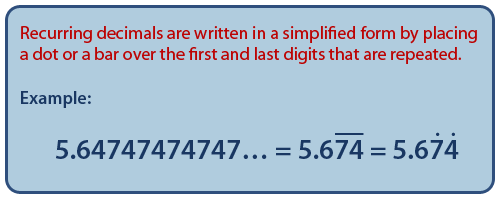All recurring decimals (see also notes on Surds and Irrational Numbers) can be written as fractions.  Some are ones you may already be familiar with already, such as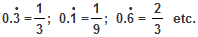In order to change the decimals into fractions, we need to use a bit of algebra.  We will begin by looking at the most common recurring decimal: 0.Let x = 0., then multiplying by 10, makes 10x = 3.Now if we write out the decimals with more digits, we find:

10x = 3.3333333…
And x = 0.3333333…

We can see from this that if we subtract the two figures, the part that is after the decimal, will be eliminated.

Hence we find:10x = 3.3333333…
x = 0.3333333…
9x = 3

### Solve the equation:

 9x = 3 x = 3 9 x = 1 3

Steps to follow to make any recurring decimal into a fraction, follows the same process.

Step 1:  Let the recurring decimal you have, be equal to x.
Step 2:  Multiply the recurring decimal by a power of 10, so that the complete recurring digits are in front of the decimal point.
Step 3:  Subtract the sets of x’s you have so that you are left with a certain number of x which is equal to a number.
Step 4:  Solve the equation.

## Examples

Example 1
Find the exact fraction of 0.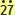Step 1: x = 0.Step 2: 100x = 27.(multiply by 100 putting the ‘27’ in front of the decimal)
Step 3: 100x = 27.x = 0.99x = 27
Step 4:
 x = 27 , which simplifies to x  = 3 . 99 11

Example 2
Find the exact fraction of 0.Step 1: x = 0.Step 2: 10x = 5.Step 3: 9x = 5
Step 4:
 x = 5 9

## Further Recurring Decimals

Typically, the above are the more straightforward recurring decimals.  You will also need to know how to deal with decimals where the digits do not recur immediately after the decimal point, e.g.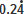,,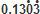etc.

You still need to follow the above steps, but just be aware of which sets of x’s you are actually subtracting.

Example 3
Find the exact fraction of 0.2Step 1: x = 0.2Step 2: 10x = 2.and 100x = 24.Step 3: 90x = 22
Note: the xparts we need to subtract is where we can eliminate the recurring decimal, after the point, i.e. 100x − 10x, if we use x, we will still end up with recurring digits after the decimal point!
Step 4:
 x = 22 , which simplifies to x  = 11 . 90 45

Example 4
Find the exact fraction of 0.13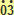Step 1: x = 0.13Step 2: 10x = 1.3and thus 10000x = 1303.3Step 3: 9990x = 1302
Step 4:
 x = 1302 = 217 9990 1665

Note: When numbers are this large, you don’t need to worry about not having simplified fully, as you are unlikely to see questions like this in the actual exam.